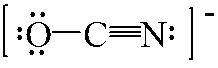# Problem: One possible structure for OCN – ion is drawn below. What is the formal charge on each atom? a. O atom = 0, C atom = 0, and N atom = 0b. O atom = 0, C atom = 0, and N atom = –1c. O atom = –1, C atom = 0, and N atom = 0d. O atom = –1, C atom = –1, and N atom = +1e. O atom = +1, C atom = 0, and N atom = –2

###### FREE Expert Solution

Use the formula of formal charges to determine the charge in each atom in OCN-

The formal charge can be calculated as:

82% (221 ratings)###### Problem Details

One possible structure for OCN ion is drawn below. What is the formal charge on each atom?a. O atom = 0, C atom = 0, and N atom = 0

b. O atom = 0, C atom = 0, and N atom = –1

c. O atom = –1, C atom = 0, and N atom = 0

d. O atom = –1, C atom = –1, and N atom = +1

e. O atom = +1, C atom = 0, and N atom = –2